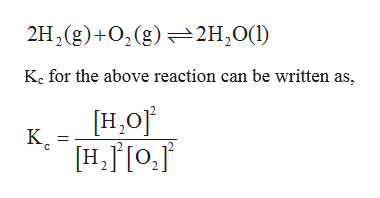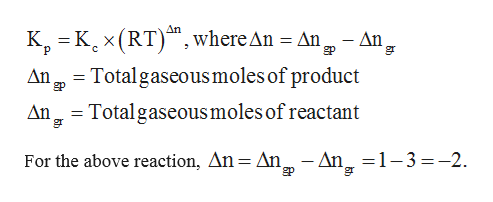# Consider the following chemical equilibrium:+2H2 (g) + O2g ⇌ 2H2OlNow write an equation below that shows how to calculate Kp from Kc for this reaction at an absolute temperature T. You can assume T is comfortably above room temperature. If you include any common physical constants in your equation be sure you use their standard symbols, found in the ALEKS Calculator.

Question
1187 views

Consider the following chemical equilibrium:

+2H2 (g) + O2g ⇌ 2H2Ol

Now write an equation below that shows how to calculate Kp from Kc for this reaction at an absolute temperature T. You can assume T is comfortably above room temperature. If you include any common physical constants in your equation be sure you use their standard symbols, found in the ALEKS Calculator.

check_circle

Step 1

The equation ishelp_outlineImage Transcriptionclose2H2(g)+0,(g) 2H20( Kc for the above reaction can be written as, H,0 К. H,o, fullscreen
Step 2

The relationship between Kp and Kc i...help_outlineImage TranscriptioncloseK Kx(RT), where An An, AnTotalgaseousmoles of product An An gr p c Total gaseous moles of reactant An An 1-3-2 gr For the above reaction, An=An, fullscreen

### Want to see the full answer?

See Solution

#### Want to see this answer and more?

Solutions are written by subject experts who are available 24/7. Questions are typically answered within 1 hour.*

See Solution
*Response times may vary by subject and question.
Tagged in

### Chemistry